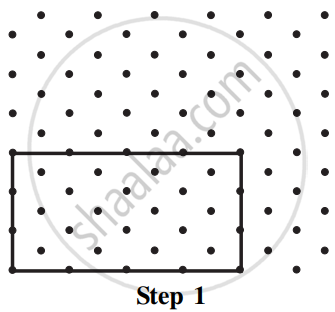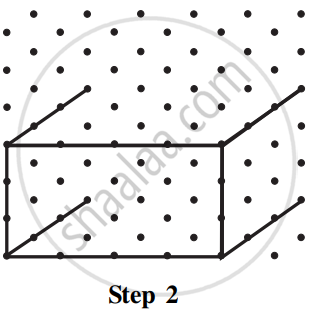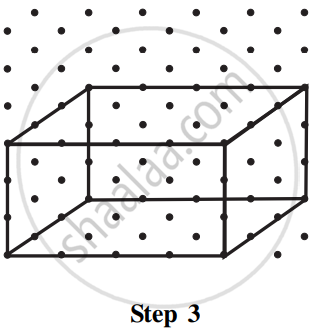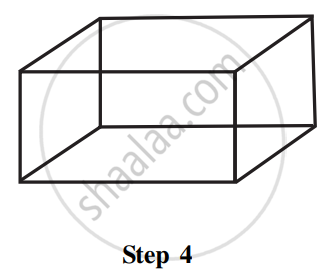Isometric Sketches:

An isometric sketch is drawn on an isometric dot paper, a sample of which is given at the end of this book. In an isometric sketch of the solid, the measurements kept proportional.

Let us attempt to draw an isometric sketch of a cuboid of dimensions 4 × 3 × 3 (which means the edges forming length, breadth, and height are 4, 3, 3 units respectively).

Step 1: Draw a rectangle to show the front face.Step 2: Draw four parallel line segments of length 3 starting from the four corners of the rectangle.Step 3: Connect the matching corners with appropriate line segments.Step 4: This is an isometric sketch of the cuboid.Note that the measurements are of exact size in an isometric sketch; this is not so in the case of an oblique sketch.

If you would like to contribute notes or other learning material, please submit them using the button below.

Shaalaa.com

Isometric Sketches [00:07:31]
S
0%